# 1. 了解 `Stan`

Stan是一种新式的语言，它提供了一种更全面的学习和实现贝叶斯模型的方法，可以适应复杂的数据结构。Stan开发团队的一个目标是通过清晰的语法、更好的采样器（这里的采样是指从贝叶斯后验分布中抽取样本）以及与许多平台（包括R、RStudio、ggplot2和Shiny）的集成，使贝叶斯建模更易于使用。

• 设计你的模型。
• 选择先验
• 对后验分布进行采样。
• 检查模型收敛（traceplots、rhats ）
• 使用后验预测批判性地评估模型并检查它们与您的数据的比较情况
• 重复…

R语言中RStan贝叶斯层次模型分析示例

# 2. 数据

```# 添加stringsAsFactors = F意味着数字变量将不会被
# 作为因子/分类变量读入
ece <- red.cv("sv", stinsAsFators = F)```

`plot( th ~ yr, data)`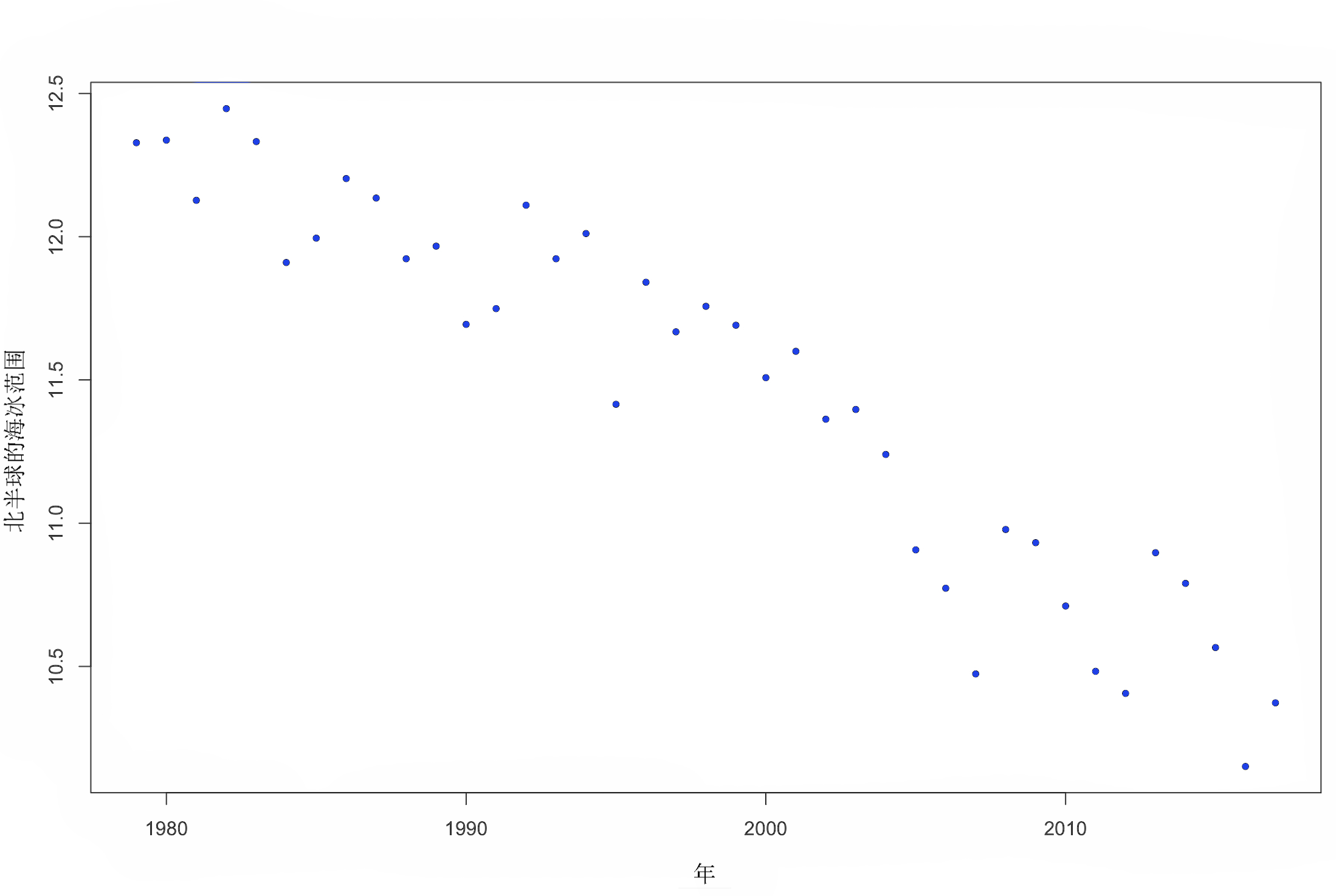图 1. 北半球海冰范围随时间的变化。

```l1 <- lm(exnoh ~ yer, data = sie)
summary(l1)```

`ablne(m1, l = 2, ty = 2, w = 3)`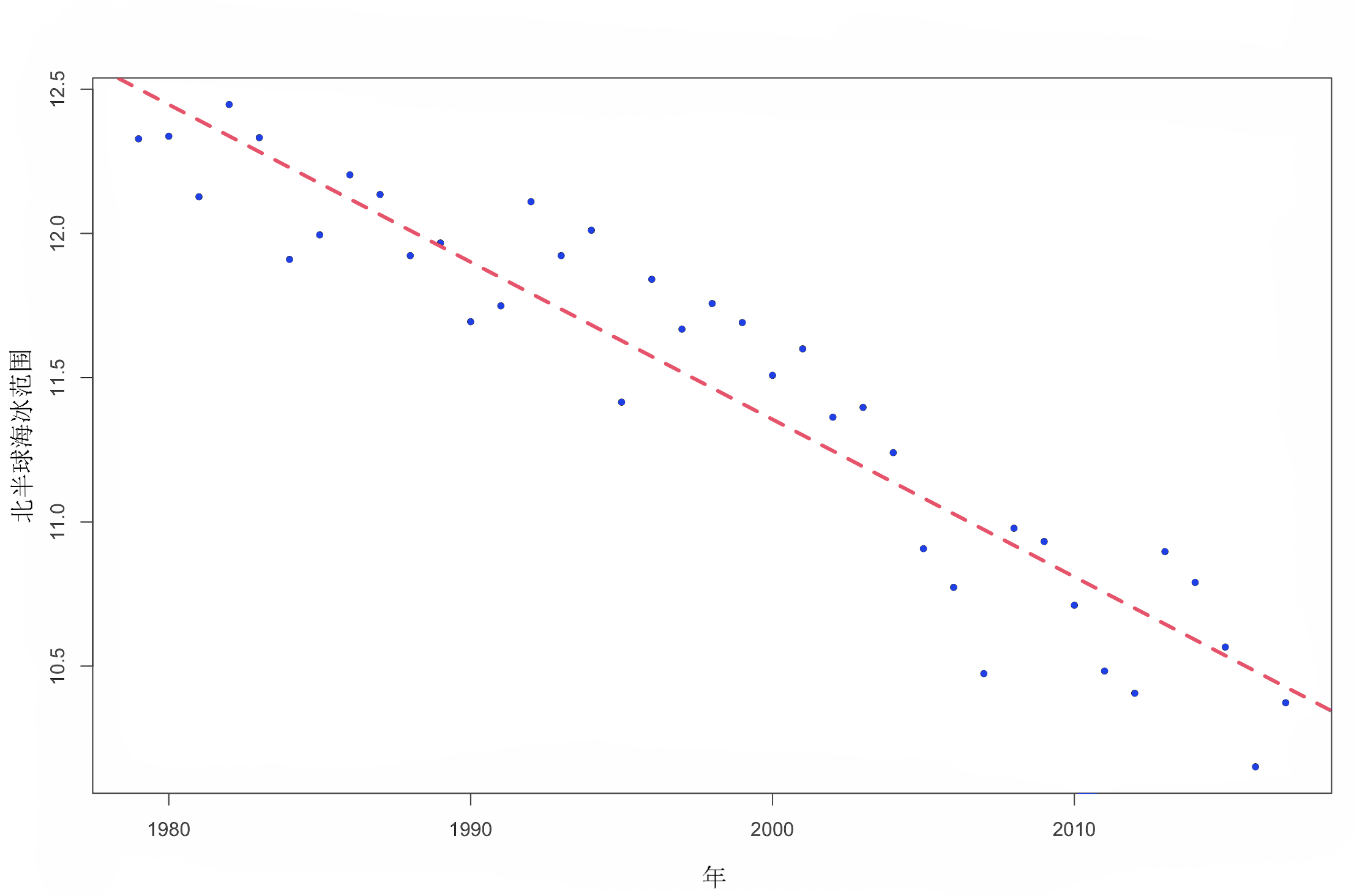图 2. 北半球海冰范围随时间的变化（加上线性模型拟合）。

y = α + β ∗ x + 误差

## 准备数据

`x <- I(year - 1978)`

`summary(lm1)`

```coeff\[1\] # 截距值
coeff\[2\] # 斜率
sigma(lm1) # 残差```

# 3. `Stan` 程序

• “数据” 块：您可以在其中声明数据类型、它们的维度、任何限制（即 upper = 或 lower = ，用作检查 `Stan`）及其名称。
• “参数” 块：您可以在此处指明要建模的参数和名称。对于线性回归，我们希望对回归线周围的误差的截距、任何斜率和标准偏差进行建模。
• “模型” 块：这是包含任何抽样语句的地方，包括正在使用的模型。模型块是指明要为参数包含的任何先验分布的地方。如果未定义`Stan` 先验，则 使用默认先验 `uniform(-infinity, +infinity)`。您可以在声明参数时使用上限或下限来限制先验（即 `lower = 0`\> 确保参数为正）。

### 随时关注您喜欢的主题

• “功能”
• “转化的数据”
• “转换后的参数
• “生成的数量”

```write("//简单线性回归的模型

int < lower = 1 > N; // 样本大小
vector\[N\] x;// 预测
vecor\[N\] y;// 结果

real alha; // 截距
real beta; // 斜率(回归系数)
real < loer = 0 > sima; // 误差SD

y ~ nrmal(alpha + x * beta , siga);

// 后验预测分布" 。

"md1.stan"```

` "model1.stan"`

# 4. 运行`Stan` 模型

`Stan` 程序`C++` 在被使用之前被遵守 。这意味着在 R 可以使用模型之前需要运行 C++ 代码。为此，您必须 `C++` 安装编译器。编译后，您可以在每个会话中多次使用模型，但在开始新`R` 会话时必须重新编译 。有许多 `C++` 编译器，而且它们在不同系统中通常是不同的。如果您的模型一堆错误，请不要担心。只要模型可以与该`stan()` 函数一起使用 ，它就可以正确编译。

# 如果我们想使用以前编写的 `.stan` 文件，我们在`file` 函数中使用 参数 `stan_model()` 。

`Stan` 如果`warmup =` 未指定参数，则自动使用一半的迭代作为预热 。

`stan(fie =moel1, data = data, wrmup = 500, ier = 1000, chais = 4, cres = 2, thn = 1)`

`fit`

# 模型输出展示了什么？你怎么知道你的模型已经收敛了？

`poteir <- exrat(fit)`

`extract()` 将每个参数的后验估计放入一个列表中。

`plot(y ~ x)`

`plot(y ~ x, pch = 20)`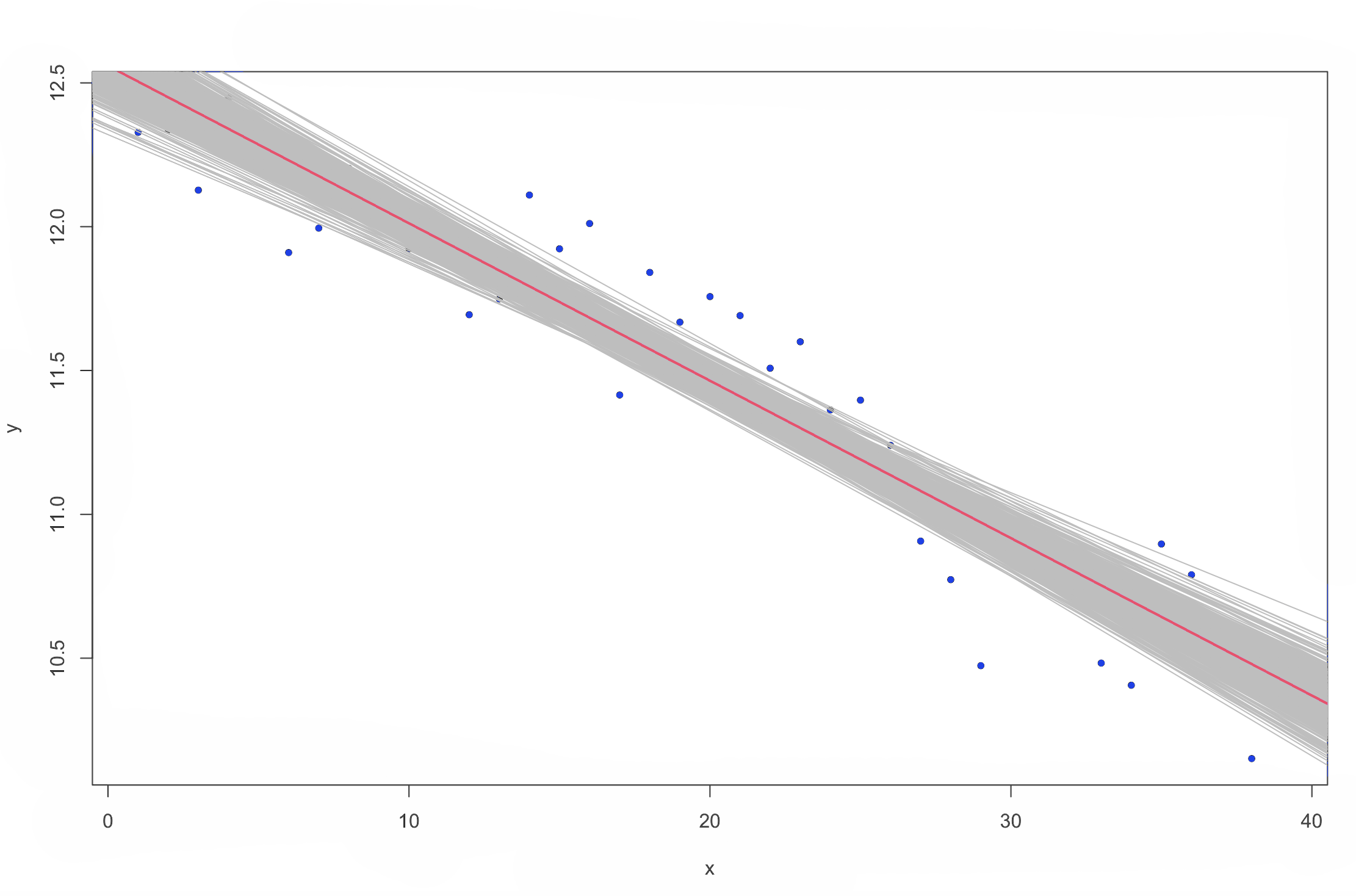图 4. 北半球海冰范围随时间的变化（`Stan` 线性模型拟合）。

# 5. 改变先验

```参数

real alha; // 截距
real bta; // 斜率(回归系数)
real < lowr = 0 > sima; // 误差SD

beta ~ nomal(1, 0.1);
y ~ normal(apha + x * beta , siga);```

``` stan(stn_oel)

plot(y ~ x)```

# 6. 收敛诊断

`n_f` 是有效样本大小的粗略度量。通常只需要担心这个数字小于迭代次数的 1/100 或 1/1000。

```plot(alpha, type = "l")
plot(beta, type = "l")
plot(sigma, type = "l")```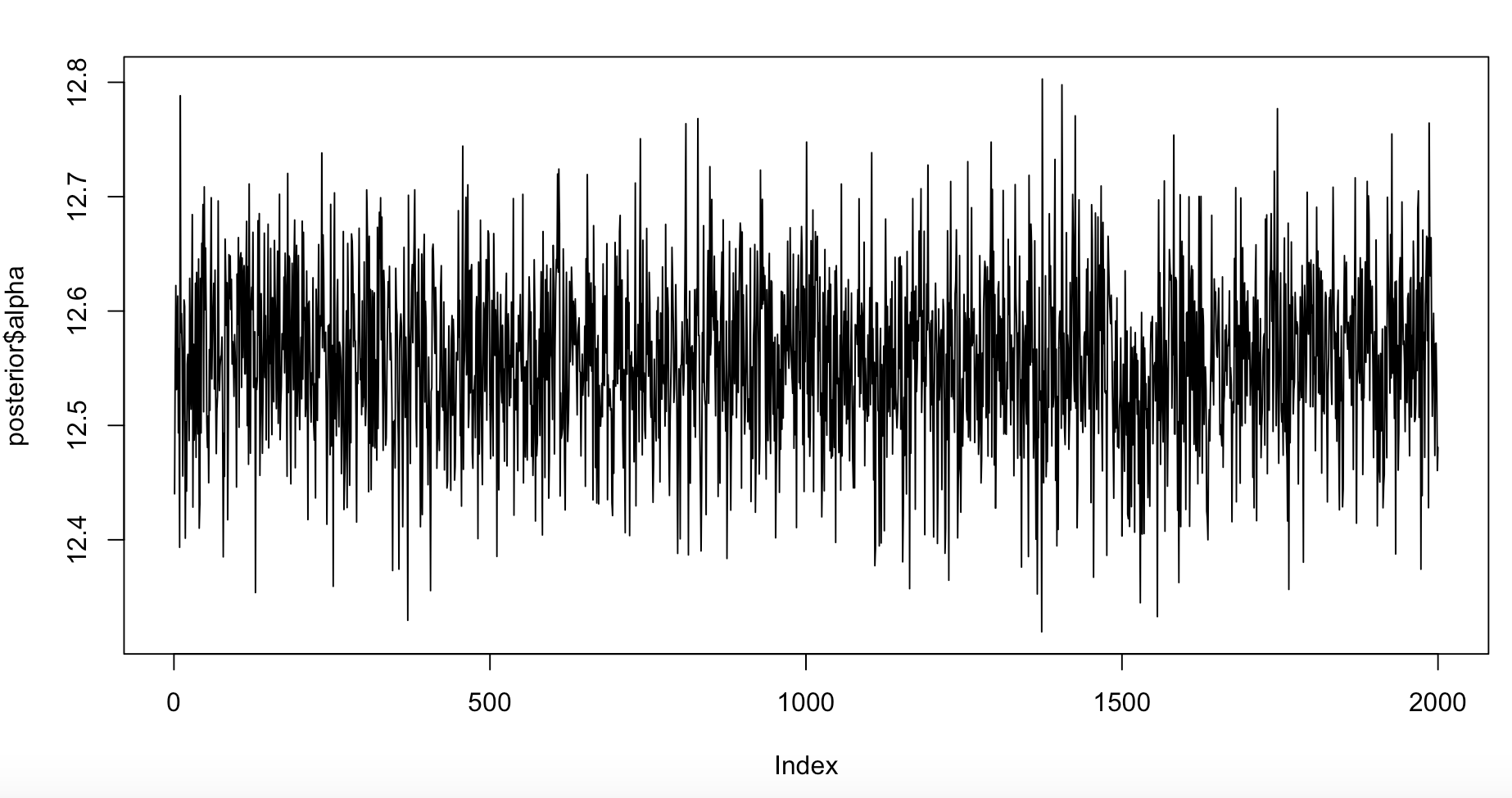图 6. alpha 的迹线图，截距。

## 收敛性差

`fid <- stan( iter = 50)`

```plot(alpha, type = "l")
plot(beta, type = "l")
plot(sigma, type = "l")```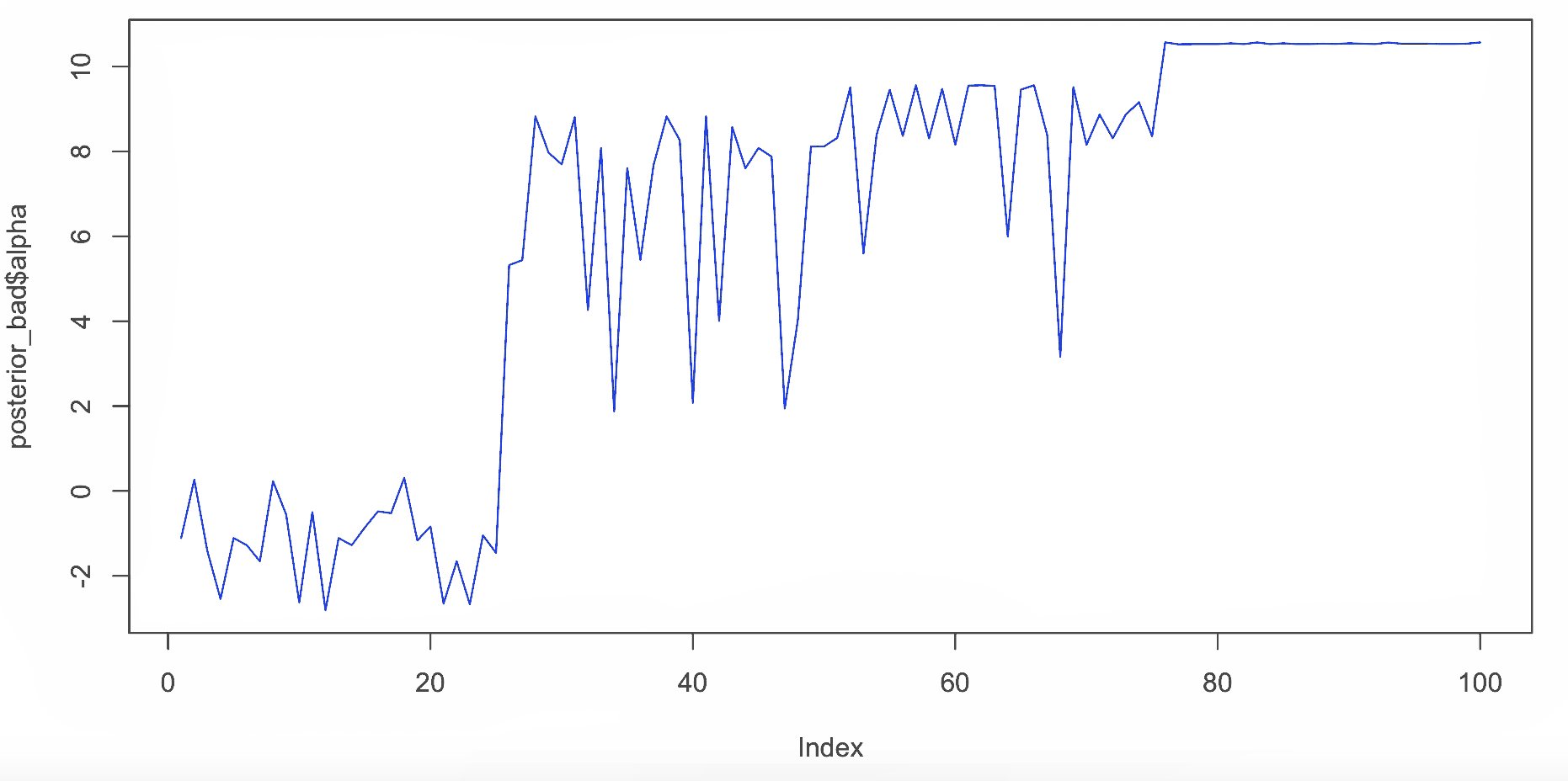图 7. alpha 的错误轨迹图，截距。

## 参数汇总

```par(mfrow = c(1,3))

plot(dnsty(alpha)```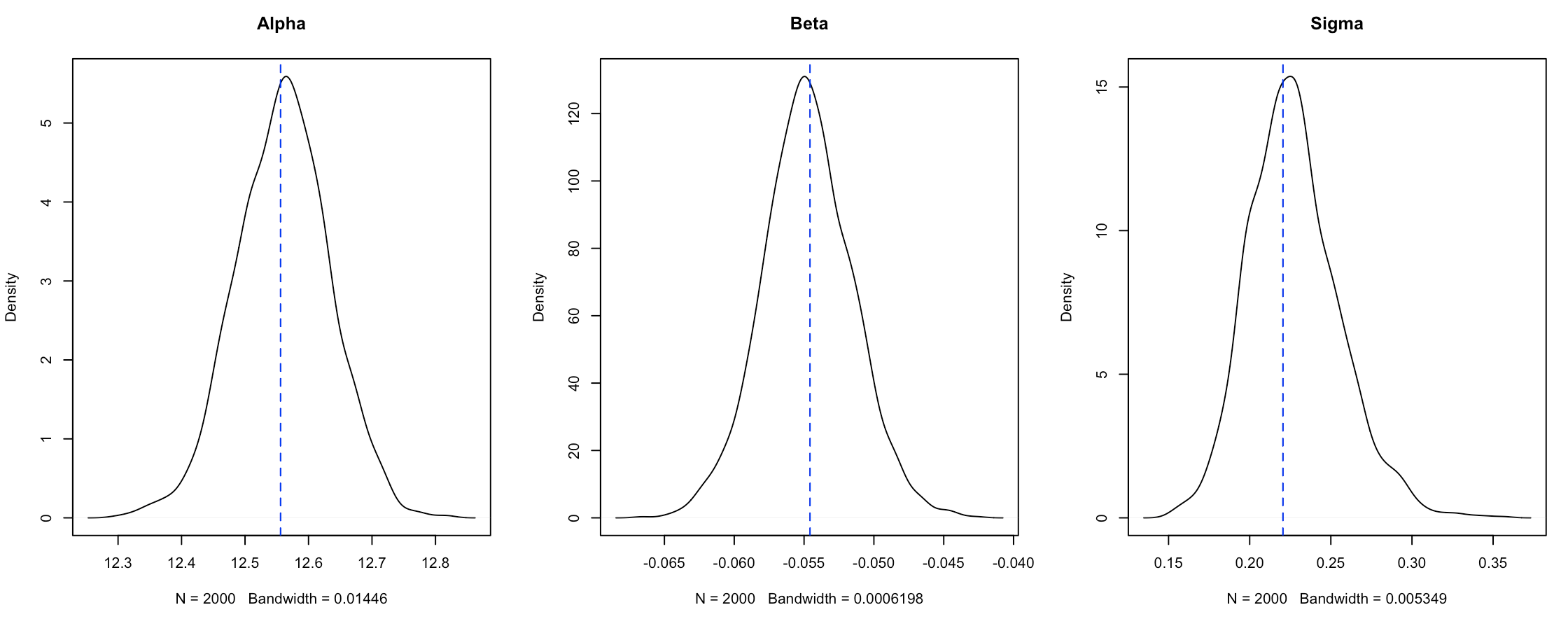图 8.`Stan` 模型拟合的密度图分布 与一般`lm` 拟合的估计值比较 。

beta > 0 的概率：

```sum(beta>0)/lenth(beta)
# 0```

beta > 0.2 的概率：

```sum(bea>0.2)/legth(beta)
# 0```

## 诊断图 `rstan`

`plot(fit)`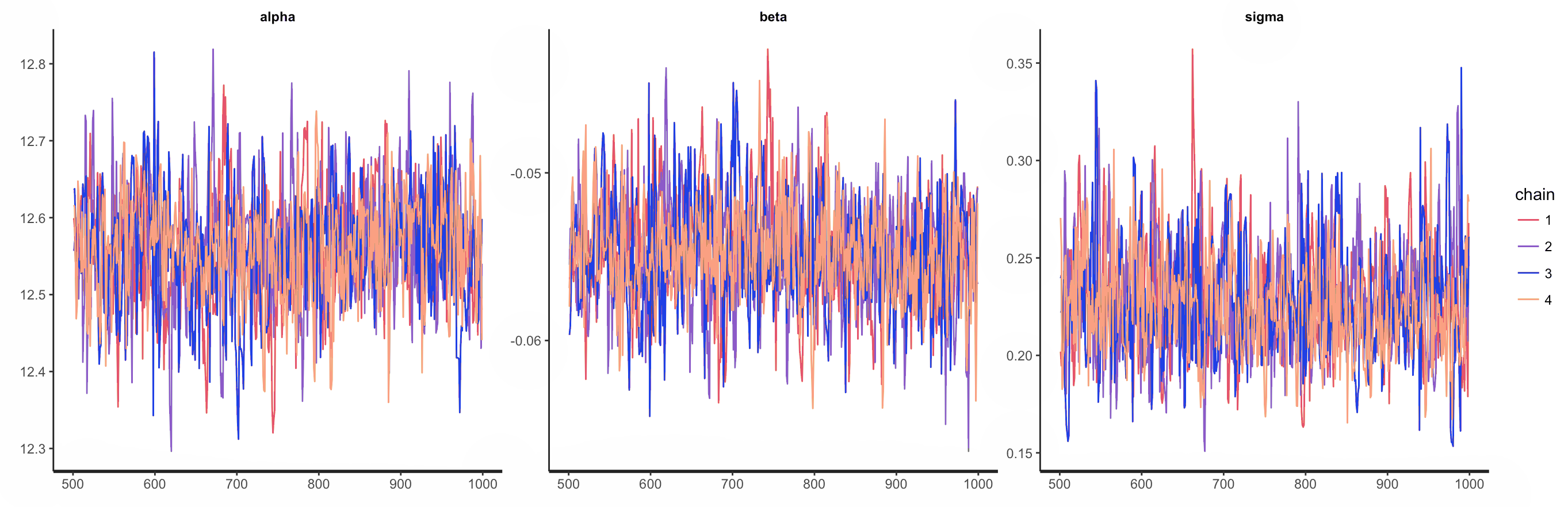图 9.`Stan` 模型不同链的跟踪图 。

```dens(it)
hist(ft)```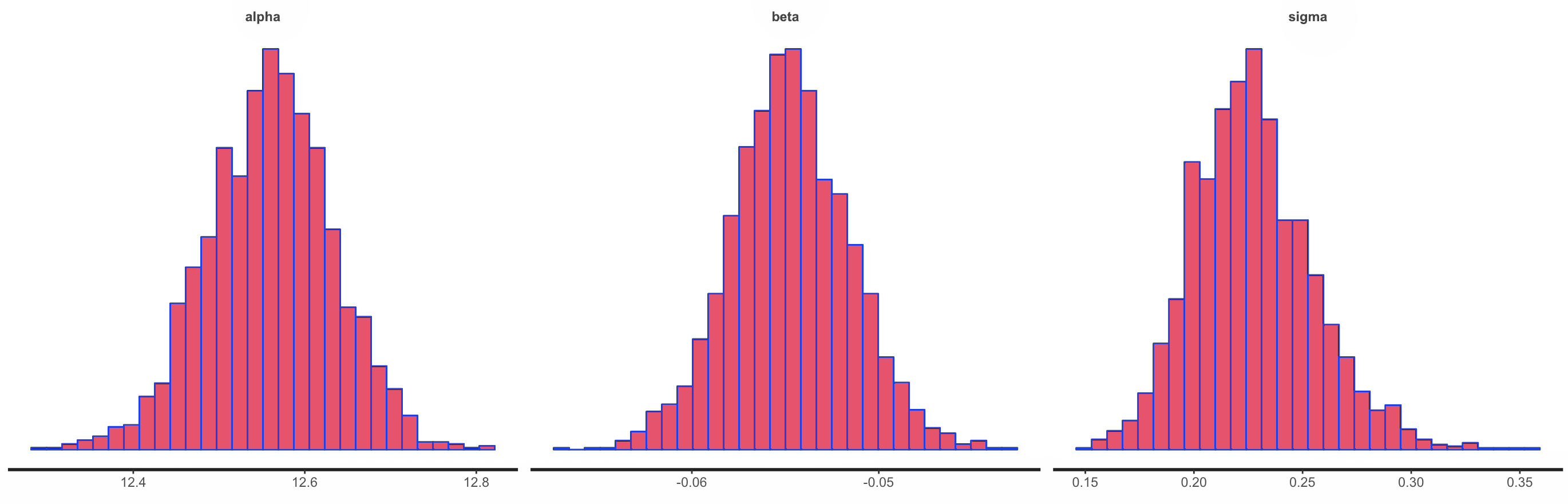图 10.`Stan` 模型中截距、斜率和残差方差的后验密度图和直方图 。

`plot(fit)`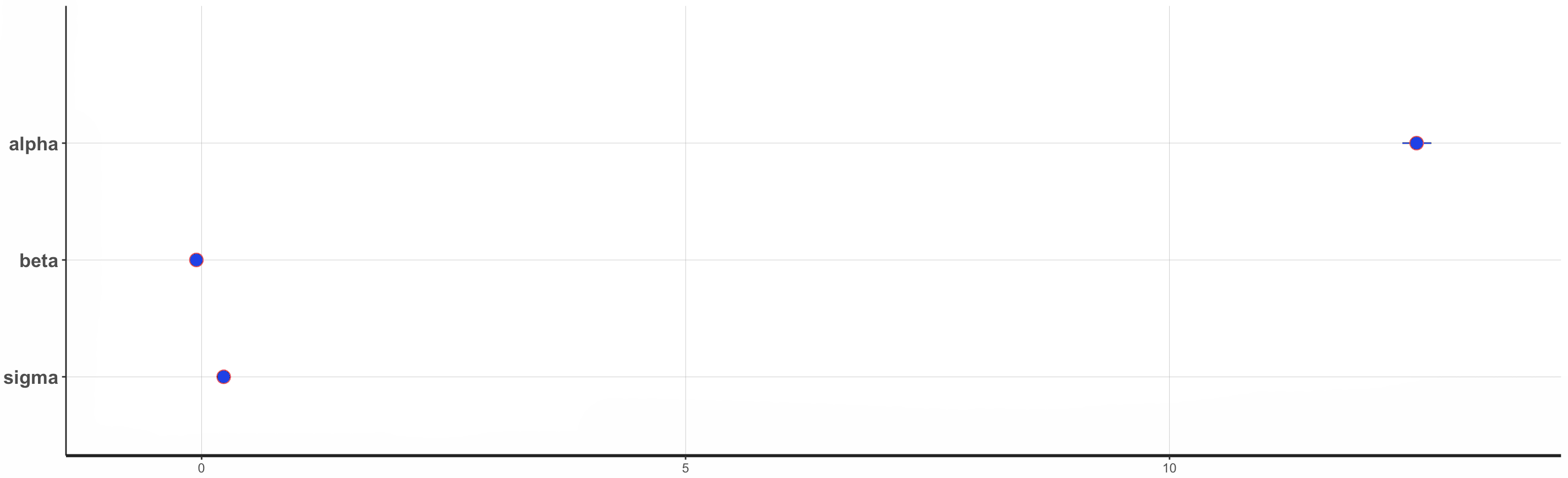图 11.`Stan` 模型的参数估计 。

## 后验预测检查

```参数

real alpha; // 截距
real beta; // 斜率(回归系数)

y ~ nomal(x * eta + alpa, sgma);

for (n in 1:N) {
yp\[n\] = normlrg(x\[n\] * bta + apha, sima) 。
}```

` stan(modl2GQ, data , ier = 1000, hans = 4, cres = 2, tin = 1)`

## `y_rep` 从后验中提取 值。

`poy(y, yrep\[1:200, \])`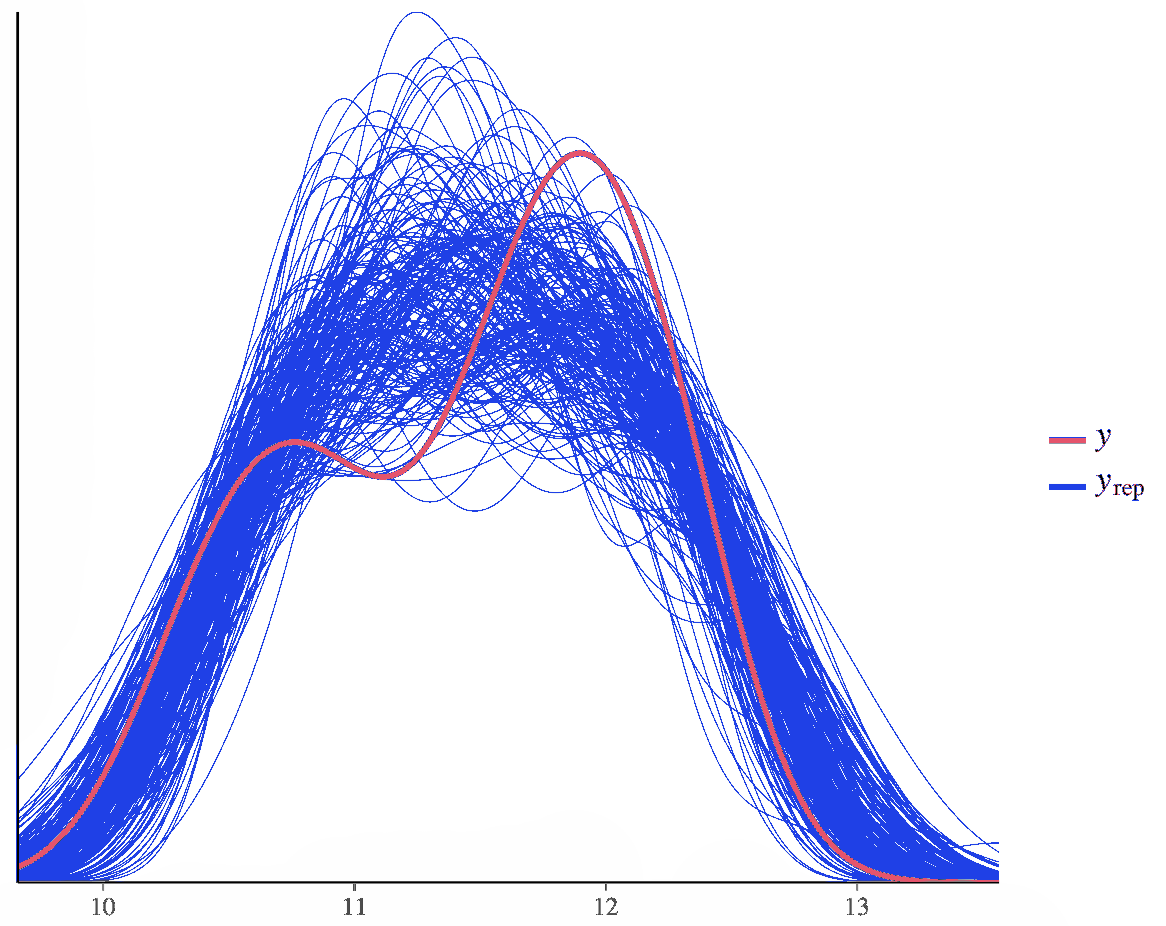图 12. 比较随机后验抽取的估计值。

`pp(y = y, yep = yrep, tat = "mean")`

`scttrg(y = y, yrp = yrep)`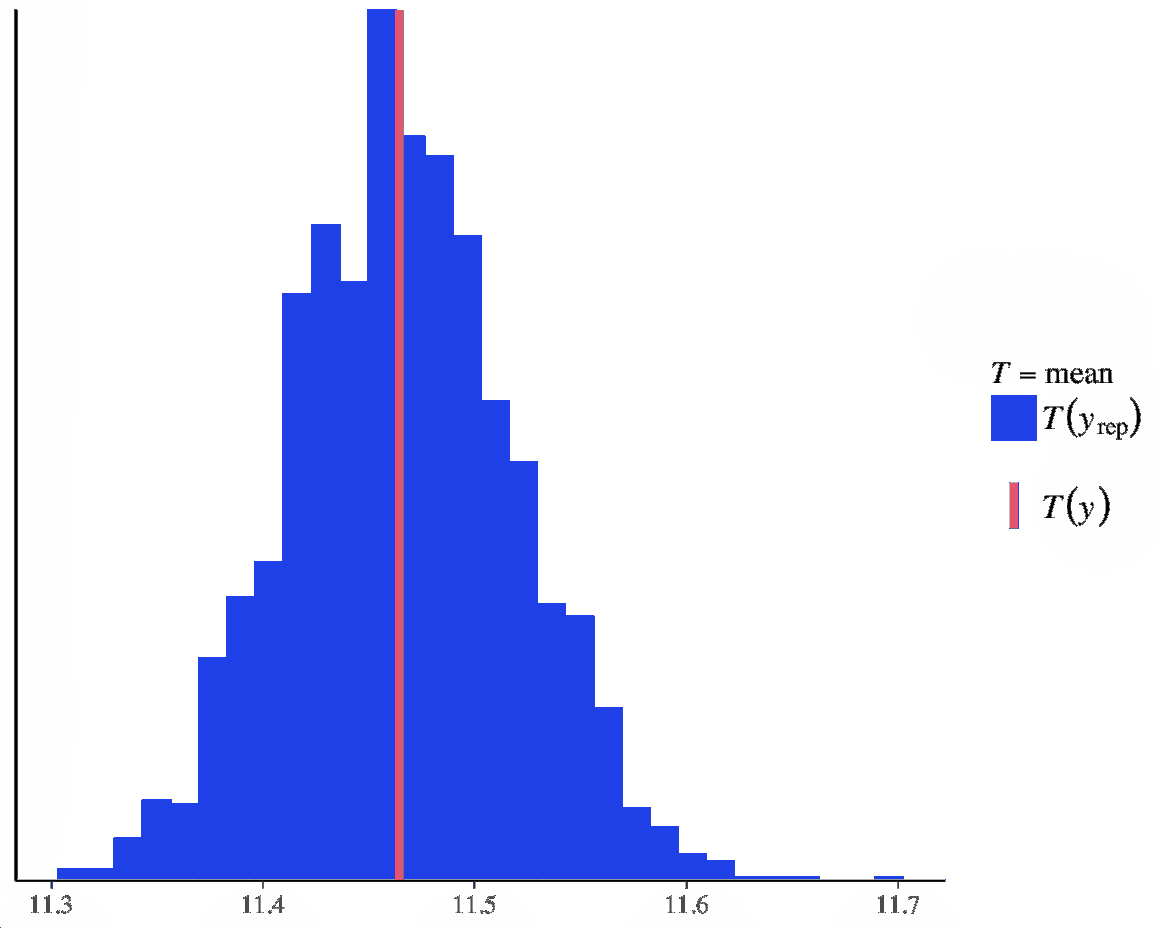图 14. 每个数据点的平均后验预测与每个数据点的观测值。

Kaizong Ye拓端研究室（TRL）的研究员。

​非常感谢您阅读本文，如需帮助请联系我们！QQ在线咨询

15121130882

0571-63341498

## 关注有关新文章的微信公众号

This will close in 0 seconds